# What is the significance of ‘Mass Excess’?

Mass Excess for an atomic nuclide is the difference between its actual mass ($m$) and its mass number ($A$) in the unified atomic mass unit ($u$). If we denote the mass excess as $\delta m_u$, then, $\boxed{ \delta m_u=(m-A)u}$. It is not the same as the related concept, mass defect in Nuclear Physics.

Mass Excess of a nuclide provides an easy estimation of its nuclear stability in comparison to the standard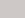$^{12} _6 C$ nuclide in terms of nuclear binding energy per nucleon (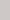$\frac{B}{A}$). Mass Excess is not the same as Mass Defect but it serves a similar purpose. Greater the mass excess less stable is the nuclide.

The mass excess of the standard$^{12} _6 C$ atom is zero by definition, since, its mass is $12 \ u$ and its mass number is $12$.

Let’s look at two usage examples of mass excess. In the first one, we shall see how the concept of mass excess can be used to easily estimate the stability of an atomic nuclide with respect to the standard$^{12} _6 C$ nuclide and in the second one, we shall see how the feasibility of a nuclear reaction can be determined using the concept.

## Example 1:

Take the standard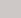$^1 _1 H$ and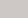$^4 _2 He$ atoms for example. Let’s find which nucleus is more stable.

The atomic mass for$^1 _1 H$ is $m=1.007825 \ u$. Its mass number $A=1$. Thus the mass excess, $\delta m_u=1.007825-1=0.007825 \ u$. We can also convert this in the unit of electronvolts by using the conversion formula, $1 \ u \approx 931.5 \ MeV/c^2$. Thus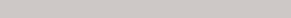$\delta m_u (^1 _1 H)=931.5 \times 0.007825 \approx \ 7.3 MeV$.

The atomic mass for$^4 _2 He$ is $m=4.002602 \ u$. Its mass number $A=4$. Thus the mass excess, $\delta m_u=4.002602-4=0.002602 \ u \approx 2.4 \ MeV$.

By comparing the mass excesses of the two we can say that$^4 _2 He$ has greater stability than$^{1}_{1}H$.

## Example 2:

Take the following nuclear reaction.

$$^{236} U \rightarrow ^{92}Kr + ^{141}Ba + 3n$$

Mass excess for $^{236}U$ is $0.045563 \ u$. For $^{92}Kr$, $^{141}Ba$, and $n$ it is $-0.073843 \ u$,$-0.085588 \ u$, and $0.008665 \ u$ respectively. When the total mass excess is calculated for the products, it comes out to be $-0.133437 \ u$. Thus we can conclusively state that the nuclear reaction is feasible since it leads to a more stable state.